Why does the following code add another line to the arc?

``````dim height as Double = 50
dim curve as Double = 50
dim offset as Double = 20
g.PenSize = 2
dim HalfPen as Double = g.PenSize/2
Const Pi = 3.14159

Dim p As New GraphicsPath
p.MoveToPoint(HalfPen, height + HalfPen)
p.AddLineToPoint(offset + HalfPen, height + HalfPen)
p.AddArc(offset + HalfPen, height + HalfPen - curve, curve, 0, Pi/2, False)
g.DrawPath(p, False)
``````

The result should be a horizontal line and an arc. But I get an additional line:

How can I remove the line in red in the screenshot above?

Try:

``````p.AddArc(offset + HalfPen, height + HalfPen - curve, curve, Pi/2, Pi/8, True)
``````

Edit: maybe is 3*Pi/2 instead of Pi/8

The code in the language references makes an arc that is identical to mine. So 0 as start angle and Pi/2 as final angle are okay:

``````Var arc As New GraphicsPath
arc.AddArc(50, 50, 20, 0, Pi / 2, False)
g.DrawPath(arc)
``````

Explanation, this code:

``````p.MoveToPoint(HalfPen, height + HalfPen)
``````

set the point here: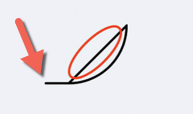this code:

``````p.AddLineToPoint(offset + HalfPen, height + HalfPen)
``````

draws a line to this point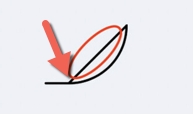this code

``````p.AddArc(offset + HalfPen, height + HalfPen - curve, curve, 0, Pi/2, False)
``````

tells Xojo to Add an Arc starting here: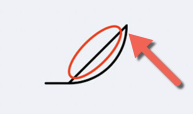and ending here:In other words, the line that you don’t want connects the end of the Line to the Start of the Arc.

Nope, that code starts an arc to the top right (0) and ends at the bottom left (pi/2).

It looks ok because there is no other reference or line connecting to it before you add that arc.

Here is the relevant part of the docs for AddArc:

x The x-coordinate of the center point of the arc.
y The y-coordinate of the center point of the arc.
startAngle The angle (in radians) that determines the starting point of the arc, measured from the x-axis.
endAngle The angle (in radians) that determines the ending point of the arc, measured from the x-axis.
clockwise A Boolean value that specifies whether or not to draw the arc in the clockwise direction.

startAngle is 0, engAngle is Pi/2. So the arc is drawn upwards and not downwards. And I still don’t know how to get rid of the line.

Maybe the docs are not clear enough. You can do this test:

``````Var arc As New GraphicsPath
arc.AddArc(50, 50, 20, 0, Pi / 2, False)
g.DrawPath(arc)
``````

Result: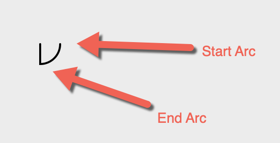I show you how, use this code:

``````Dim p As New GraphicsPath
p.MoveToPoint(HalfPen, height + HalfPen)
p.AddLineToPoint(offset + HalfPen, height + HalfPen)
p.AddArc(offset + HalfPen, height + HalfPen - curve, curve, Pi/2, 0, True)
g.DrawPath(p, False)
``````

and get this: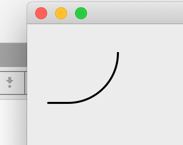Edit: I made a mistake it is 0 to create a 90 degree arc. 3*Pi/2 will create a 180 degree arc (my test cut the other 90 degree arc).

Thanks! I don’t get GraphicsPath, really.

Glad I was able to help.

It took me some time to understand that the graphics are upside/down, so the arc to create a circle is like this:I edited the code to change 3*pi/2 to 0. You wanted to create an Arc from 90 to 0 but your code said to draw it from 0 to 90 that is why you got a ‘connecting’ line.

Also, the clockwise boolean is backward as we normally use because the graphics are flipped, so an arc from 90 to 0 that is counter-clockwise for us is clockwise for the graphic path.

I hope this information helps to make sense on how to use the arc.

This thing doesn’t make any sense at all. Yes, the image helps. That’s why I got so confused.